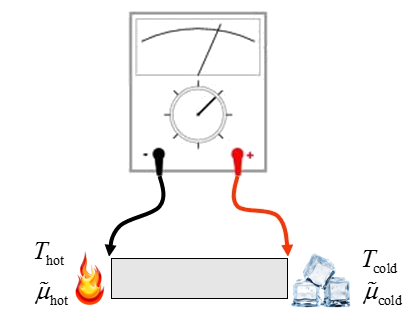## Seebeck Effect

The Seebeck effect relates the gradient of the electrochemical potential to the temperature gradient.

\begin{equation} \nabla_{\vec{r}}\tilde{\mu}= -eS\nabla_{\vec{r}}T. \end{equation}

Here $e$ is the elementary charge and $S$ is the Seebeck coefficient in units of V/K. The Seebeck coefficient is also known as the thermopower. If one side of a material is hot and the other is cold, the charge carriers will diffuse more from hot to cold. If the hot and cold ends are not connected electrically, a charge and concentration gradient is establish that opposes the diffusion caused by the temperature difference. A voltmeter would measure a voltage difference $(\tilde{\mu}\text(\text{cold})-\tilde{\mu}(\text{hot}))/e$.Generally, the Seebeck coefficient is a matrix,

\begin{equation} \left[ \begin{array}{cccc} \frac{\partial \tilde{\mu}}{\partial x} \\ \frac{\partial \tilde{\mu}}{\partial y} \\ \frac{\partial \tilde{\mu}}{\partial z} \\ \end{array} \right]=-e \left[ \begin{array}{cccc} S_{xx} & S_{xy} & S_{xz} \\ S_{yx} & S_{yy} & S_{yz} \\ S_{zx} & S_{zy} & S_{zz} \\ \end{array} \right]\left[ \begin{array}{cccc} \frac{\partial T}{\partial x} \\ \frac{\partial T}{\partial y} \\ \frac{\partial T}{\partial z} \\ \end{array} \right]. \end{equation}

The elements of the Seebeck matrix can be derived from the general expression for the electronic current density is,

\begin{equation} \vec{j}_{\text{elec}}= \frac{e}{4\pi^3\hbar^2}\int \tau (\vec{k}) \frac{\partial f_0}{\partial \mu}\nabla_{\vec{k}}E(\vec{k})\left( \nabla_{\vec{k}}E(\vec{k})\cdot\left(\nabla_{\vec{r}}\tilde{\mu}+\frac{E(\vec{k})-\mu}{T}\nabla_{\vec{r}}T \right) \right) d^3k. \end{equation}

For the experiment we are considering, the ends are not electrically connected so no current flows, $\vec{j}_{\text{elec}} = 0$,

\begin{equation} 0 = \frac{e}{4\pi^3\hbar^2}\int \tau (\vec{k}) \frac{\partial f_0}{\partial \mu}\nabla_{\vec{k}}E(\vec{k})\left( \nabla_{\vec{k}}E(\vec{k})\cdot\left(\nabla_{\vec{r}}\tilde{\mu}+\frac{E(\vec{k})-\mu}{T}\nabla_{\vec{r}}T \right) \right) d^3k. \end{equation}

This can be expressed as 9 equations which can solved for the elements of the Seebeck matrix,

\begin{equation} 0 = \frac{e}{4\pi^3\hbar^2}\int \tau (\vec{k}) \frac{\partial f_0}{\partial \mu}\nabla_{\vec{k}}E(\vec{k})\cdot\hat{e}_i\left( \nabla_{\vec{k}}E(\vec{k})\cdot\left(\left( \begin{array}{cccc} -eS_{xj} \\ -eS_{yj} \\ -eS_{zj} \end{array} \right)+\frac{E(\vec{k})-\mu}{T} \right) \hat{e}_j \right) d^3k. \end{equation}

To solve these equations we choose the temperature gradient to be a unit vector. If $\nabla T= \hat{x}$ then $\nabla\tilde{\mu}=-eS_{xx}\hat{x}-eS_{yx}\hat{y}-eS_{zx}\hat{z}$. We have three equations to solve for these three coefficients since each component of $\vec{j}_{\text{elec}}$ must be zero. Typically such equations are solved numerically by guessing the Seebeck coefficients and testing if $\vec{j}_{\text{elec}}=0$ and then iterating.

If the crystal is cubic, then the Seebeck coefficient is a scalar,

\begin{equation} 0 = \frac{e}{4\pi^3\hbar^2}\int \tau (\vec{k}) \frac{\partial f_0}{\partial \mu}\nabla_{\vec{k}}E(\vec{k})\cdot\hat{z}\left( \nabla_{\vec{k}}E(\vec{k})\cdot\left(-eS+\frac{E(\vec{k})-\mu}{T} \right) \hat{z} \right) d^3k. \end{equation}

In this case the Seebeck coefficient can be written as the ratio of two integrals,

\begin{equation} eS = -\frac{\int \tau (\vec{k}) \frac{\partial f_0}{\partial \mu}\nabla_{\vec{k}}E(\vec{k})\cdot\hat{z}\left( \nabla_{\vec{k}}E(\vec{k})\cdot\left(\frac{E(\vec{k})-\mu}{T} \right) \hat{z} \right) d^3k}{\int \tau (\vec{k}) \frac{\partial f_0}{\partial \mu}\nabla_{\vec{k}}E(\vec{k})\cdot\hat{z}\left( \nabla_{\vec{k}}E(\vec{k})\cdot \hat{z} \right) d^3k}. \end{equation}

The denominator is proportional to the electrical conductivity for cubic crystals so we can write this as,

$$\sigma S = -\frac{e}{3\pi^2}\int\limits_0^{\infty} \tau (\vec{k}) \frac{\partial f_0}{\partial \mu}\left| \vec{v}_{\vec{k}}\right|^2 \left(\frac{E(\vec{k})-\mu}{T} \right) k^2 dk .$$

Comparing this to the result for the thermoelectric coefficient for cubic crystals we see that,

$$\sigma S = -\kappa.$$

A heat current will also flow in this case. The expression for the heat current is,

\begin{equation} \vec{j}_Q= -\frac{1}{4\pi^3\hbar^2}\int \tau (\vec{k}) \frac{\partial f_0}{\partial \mu}\left( E(\vec{k}) - \mu \right)\nabla_{\vec{k}}E(\vec{k})\left( \nabla_{\vec{k}}E(\vec{k})\cdot\left(\nabla_{\vec{r}}\tilde{\mu}+\frac{E(\vec{k})-\mu}{T}\nabla_{\vec{r}}T \right) \right) d^3k. \end{equation}

In this experiment, the electrochemical potential and the temperature gradient are related by $\nabla_{\vec{r}}\tilde{\mu}= -eS\nabla_{\vec{r}}T$ so this is inserted into the expression for the heat current.

\begin{equation} \vec{j}_Q= -\frac{1}{4\pi^3\hbar^2}\int \tau (\vec{k}) \frac{\partial f_0}{\partial \mu}\left( E(\vec{k}) - \mu \right)\nabla_{\vec{k}}E(\vec{k})\left( \nabla_{\vec{k}}E(\vec{k})\cdot\left(-eS\nabla_{\vec{r}}T+\frac{E(\vec{k})-\mu}{T}\nabla_{\vec{r}}T \right) \right) d^3k. \end{equation}

The thermal conductivity in this case is,

\begin{equation} K_{ij}= -\frac{1}{4\pi^3\hbar^2}\int \tau (\vec{k}) \frac{\partial f_0}{\partial \mu}\left( E(\vec{k}) - \mu \right)\nabla_{\vec{k}}E(\vec{k})\cdot\hat{e}_i\left( \nabla_{\vec{k}}E(\vec{k})\cdot\left(-eS\hat{e}_j+\frac{E(\vec{k})-\mu}{T}\hat{e}_j \right) \right) d^3k. \end{equation}

Notice that we arrived at a different expression for the electrical contribution to the thermal conductivity if the hot and cold ends are electrically shorted than if they are electrically open. More generally, the thermal conductivity will depend on the voltage drop across the material. In practice, the Seebeck coefficients are quite small (in the range of mV/K) so the voltage dependence of the thermal conductivity can be neglected.